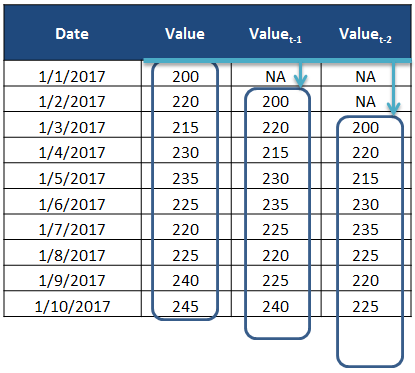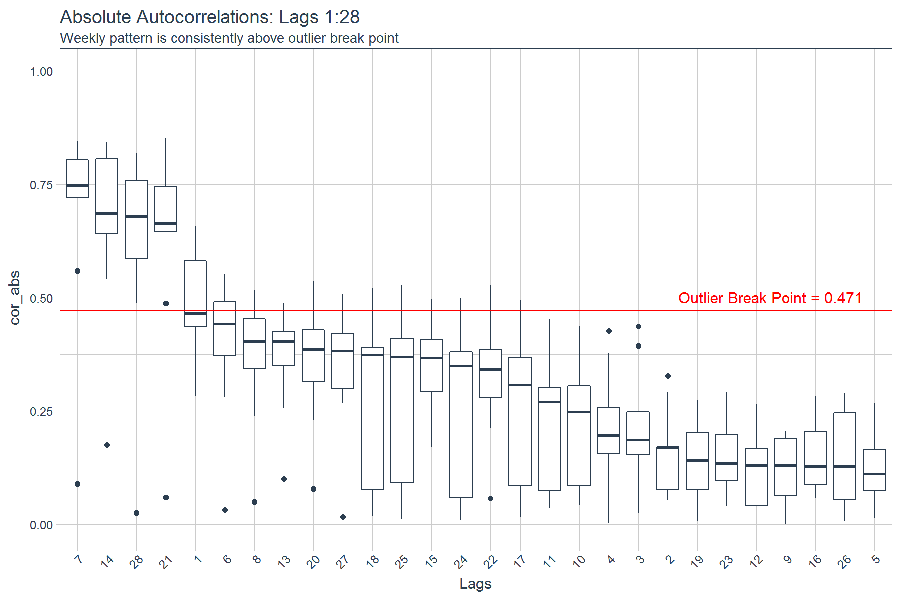Want to share your content on R-bloggers? click here if you have a blog, or here if you don't.

In the fourth part in a series on Tidy Time Series Analysis, we’ll investigate lags and autocorrelation, which are useful in understanding seasonality and form the basis for autoregressive forecast models such as AR, ARMA, ARIMA, SARIMA (basically any forecast model with “AR” in the acronym). We’ll use the tidyquant package along with our tidyverse downloads data obtained from cranlogs. The focus of this post is using lag.xts(), a function capable of returning multiple lags from a xts object, to investigate autocorrelation in lags among the daily tidyverse package downloads. When using lag.xts() with tq_mutate() we can scale to multiple groups (different tidyverse packages in our case). If you like what you read, please follow us on social media to stay up on the latest Business Science news, events and information! As always, we are interested in both expanding our network of data scientists and seeking new clients interested in applying data science to business and finance. If interested, contact us.

If you haven’t checked out the previous tidy time series posts, you may want to review them to get up to speed.

Here’s an example of the autocorrelation plot we investigate as part of this post:# Libraries Needed

We’ll need to load several libraries today.

We’ll be using the same “tidyverse” dataset as the last several posts. The script below gets the package downloads for the first half of 2017.

We can visualize daily downloads, but detecting the trend is quite difficult due to noise in the data.# Lags (Lag Operator)

The lag operator (also known as backshift operator) is a function that shifts (offsets) a time series such that the “lagged” values are aligned with the actual time series. The lags can be shifted any number of units, which simply controls the length of the backshift. The picture below illustrates the lag operation for lags 1 and 2.Lags are very useful in time series analysis because of a phenomenon called autocorrelation, which is a tendency for the values within a time series to be correlated with previous copies of itself. One benefit to autocorrelation is that we can identify patterns within the time series, which helps in determining seasonality, the tendency for patterns to repeat at periodic frequencies. Understanding how to calculate lags and analyze autocorrelation will be the focus of this post.

Finally, lags and autocorrelation are central to numerous forecasting models that incorporate autoregression, regressing a time series using previous values of itself. Autoregression is the basis for one of the most widely used forecasting techniques, the autoregressive integrated moving average model or ARIMA for short. Possibly the most widely used tool for forecasting, the forecast package by Rob Hyndman, implements ARIMA (and a number of other forecast modeling techniques). We’ll save autoregression and ARIMA for another day as the subject is truly fascinating and deserves its own focus.

# Background on Functions Used

The xts, zoo, and TTR packages have some great functions that enable working with time series. The tidyquant package enables a “tidy” implementation of these functions. You can see which functions are integrated into tidyquant package using tq_mutate_fun_options(). We use glimpse() to shorten the output.

#### lag.xts()

Today, we’ll take a look at the lag.xts() function from the xts package, which is a really great function for getting multiple lags. Before we dive into an analysis, let’s see how the function works. Say we have a time series of ten values beginning in 2017.

The lag.xts() function generates a sequence of lags (t-1, t-2, t-3, …, t-k) using the argument k. However, it only works on xts objects (or other matrix, vector-based objects). In other words, it fails on our “tidy” tibble. We get an “unsupported type” error.

Now, watch what happens when converted to an xts object. We’ll use tk_xts() from the timetk package to coerce from a time-based tibble (tibble with a date or time component) to and xts object.

The timetk package is a toolkit for working with time series. It has functions that simplify and make consistent the process of coercion (converting to and from different time series classes). In addition, it has functions to aid the process of time series machine learning and data mining. Visit the docs to learn more.

We get our lags! However, we still have one problem: We need our original values so we can analyze the counts against the lags. If we want to get the original values too, we can do something like this.

That’s a lot of work for a simple operation. Fortunately we have tq_mutate() to the rescue!

#### tq_mutate()

The tq_mutate() function from tidyquant enables “tidy” application of the xts-based functions. The tq_mutate() function works similarly to mutate() from dplyr in the sense that it adds columns to the data frame.

The tidyquant package enables a “tidy” implementation of the xts-based functions from packages such as xts, zoo, quantmod, TTR and PerformanceAnalytics. Visit the docs to learn more.

Here’s a quick example. We use the select = value to send the “value” column to the mutation function. In this case our mutate_fun = lag.xts. We supply k = 5 as an additional argument.

That’s much easier. We get the value column returned in addition to the lags, which is the benefit of using tq_mutate(). If you use tq_transmute() instead, the result would be the lags only, which is what lag.xts() returns.

Now that we understand a little more about lags and the lag.xts() and tq_mutate() functions, let’s put this information to use with a lag and autocorrelation analysis of the tidyverse package downloads. We’ll analyze all tidyverse packages together, showing off the scalability of tq_mutate().

#### Scaling the Lag and Autocorrelation Calculation

First, let’s get lags 1 through 28 (4 weeks of lags). The process is quite simple: we take the tidyverse_downloads data frame, which is grouped by package, and apply tq_mutate() using the lag.xts function. We can provide column names for the new columns by prefixing “lag_” to the lag numbers, k, which the sequence from 1 to 28. The output is all of the lags for each package.

Next, we need to correlate each of the lags to the “count” column. This involves a few steps that can be strung together in a dplyr pipe (%>%):

1. The goal is to get count and each lag side-by-side so we can do a correlation. To do this we use gather() to pivot each of the lagged columns into a “tidy” (long format) data frame, and we exclude “package”, “date”, and “count” columns from the pivot.

2. Next, we convert the new “lag” column from a character string (e.g. “lag_1”) to numeric (e.g. 1) using mutate(), which will make ordering the lags much easier.

3. Next, we group the long data frame by package and lag. This allows us to calculate using subsets of package and lag.

4. Finally, we apply the correlation to each group of lags. The summarize() function can be used to implement cor(), which takes x = count and y = lag_value. Make sure to pass use = "pairwise.complete.obs", which is almost always desired. Additionally, the 95% upper and lower cutoff can be approximated by:

$$cutoff = \pm \frac{2}{N^{0.5}}$$

Where:

• N = number of observations.

Putting it all together:

#### Visualizing Autocorrelation: ACF Plot

Now that we have the correlations calculated by package and lag number in a nice “tidy” format, we can visualize the autocorrelations with ggplot to check for patterns. The plot shown below is known as an ACF plot, which is simply the autocorrelations at various lags. Initial examination of the ACF plots indicate a weekly frequency.#### Which Lags Consistently Stand Out?

We see that there appears to be a weekly pattern, but we want to be sure. We can verify the weekly pattern assessment by reviewing the absolute value of the correlations independent of package. We take the absolute autocorrelation because we use the magnitude as a proxy for how much explanatory value the lag provides. We’ll use dplyr functions to manipulate the data for visualization:

1. We drop the package group constraint using ungroup().

2. We calculate the absolute correlation using mutate(). We also convert the lag to a factor, which helps with reordering the plot later.

3. We select() only the “lag” and “cor_abs” columns.

4. We group by “lag” to lump all of the lags together. This enables us to determine the trend independent of package.

We can now visualize the absolute correlations using a box plot that lumps each of the lags together. We can add a line to indicate the presence of outliers at values above $1.5IQR$. If the values are consistently above this limit, the lag can be considered an outlier. Note that we use the fct_reorder() function from forcats to organize the boxplot in order of decending magnitude.Lags in multiples of seven have the highest autocorrelation and are consistently above the outlier break point indicating the presence of a strong weekly pattern. The autocorrelation with the seven-day lag is the highest, with a median of approximately 0.75. Lags 14, 21, and 28 are also outliers with median autocorrelations in excess of our outlier break point of 0.471.

Note that the median of Lag 1 is essentially at the break point indicating that half of the packages have a presence of “abnormal” autocorrelation. However, this is not part of a seasonal pattern since a periodic frequency is not present.

# Conclusions

Lag and autocorrelation analysis is a good way to detect seasonality. We used the autocorrelation of the lagged values to detect “abnormal” seasonal patterns. In this case, the tidyverse packages exhibit a strong weekly pattern. We saw how the tq_mutate() function was used to apply lag.xts() to the daily download counts to efficiently get lags 1 through 28. Once the lags were retrieved, we used other dplyr functions such as gather() to pivot the data and summarize() to calculate the autocorrelations. Finally, we saw the power of visual analysis of the autocorrelations. We created an ACF plot that showed a visual trend. Then we used a boxplot to detect which lags had consistent outliers. Ultimately a weekly pattern was confirmed.

We have a full suite of data science services to supercharge your organizations financial and business performance! For example, our experienced data scientists reduced a manufacturer’s sales forecasting error by 50%, which led to improved personnel planning, material purchasing and inventory management.

How do we do it? With team-based data science: Using our network of data science consultants with expertise in Marketing, Forecasting, Finance, Human Resources and more, we pull together the right team to get custom projects done on time, within budget, and of the highest quality. Learn about our data science services or contact us!

We are growing! Let us know if you are interested in joining our network of data scientist consultants. If you have expertise in Marketing Analytics, Data Science for Business, Financial Analytics, Forecasting or data science in general, we’d love to talk. Contact us!